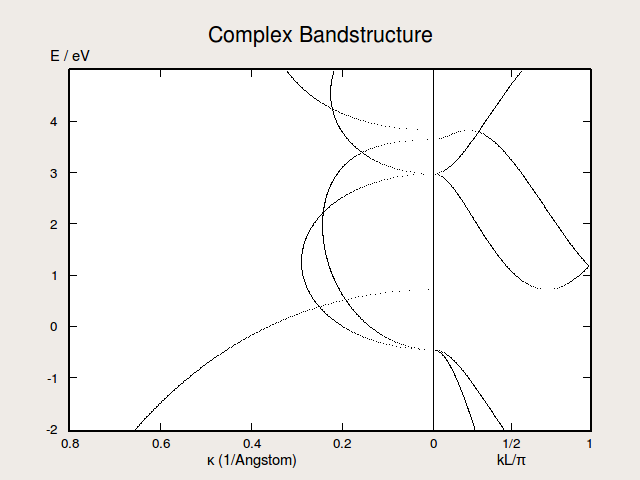# ComplexBandstructure¶

class ComplexBandstructure(configuration, energies=None, k_point=None, energy_zero_parameter=None)

Analysis class for calculating the complex bandstructure for a bulk configuration.

Parameters: configuration (BulkConfiguration) – The bulk configuration with an attached calculator for which to calculate the complex bandstructure. energies (PhysicalQuantity of type energy) – The energies for which to calculate the complex bandstructure. Default: numpy.linspace(-2, 2, 101) * eV k_point (tuple of floats) – The 2-dimensional fractional k-point (in the kA-kB plane) for which to calculate the complex bandstructure. The k-point is given as a tuple of length 2. Default: (0, 0) energy_zero_parameter (FermiLevel | AbsoluteEnergy) – Specifies the choice for the energy zero. Default: FermiLevel
energies()
Returns: The energies for which the complex bandstructure is calculated. PhysicalQuantity of type energy
energyZero()
Returns: The energy zero. PhysicalQuantity of type energy
evaluate(spin=None)

Method for obtaining the complex bandstructure for a specific spin. The complex bandstructure has two components, propagating modes and decaying modes. Both types of modes can be characterized by a value k. For propagating modes k is real, giving the Bloch phase factor in the reciprocal C-direction, whereas decaying modes have a complex value of k.

Parameters: spin (Spin.Up | Spin.Down | Spin.All) – The spin to calculate for. Default: Spin.All A tuple with two lists, the first corresponding to the propagating modes and the second corresponding to the decaying modes. The lists are ordered according to energy and each entry is a PhysicalQuantity with a (1/Angstrom) unit. The lists have different lengths (and can have zero length) corresponding to the number of modes at this energy. tuple of lists
fermiLevel(spin=None)
Parameters: spin (Spin.Up | Spin.Down | Spin.All) – The spin the Fermi level should be returned for. Must be either Spin.Up, Spin.Down, or Spin.All. Only when the band structure is calculated with a fixed spin moment will the Fermi level depend on spin. Default: Spin.All The Fermi level in absolute energy. PhysicalQuantity of type energy
fermiTemperature()
Returns: The Fermi temperature. PhysicalQuantity of type temperature
kPoint()
Returns: The 2-dimensional fractional k-koint for which the complex bandstructure is calculated. tuple of floats
layerSeparation()
Returns: The distance between layers of the crystal in the transport direction. The transport direction is perpendicular to the AB-plane. This value is called ‘L’ in the GUI. PhysicalQuantity of type length
metatext()
Returns: The metatext of the object or None if no metatext is present. str | unicode | None
nlprint(stream=None)

Print a string containing an ASCII table useful for plotting the AnalysisSpin object.

Parameters: stream (python stream) – The stream the table should be written to. Default: NLPrintLogger()
repetition()

Deprecated

setMetatext(metatext)

Set a given metatext string on the object.

Parameters: metatext (str | unicode | None) – The metatext string that should be set. A value of “None” can be given to remove the current metatext.
spins()
Returns: The available spin components. tuple of Spin.Up | Spin.Down | Spin.All

## Usage Examples¶

Calculate the complex bandstructure of silicon in the (100) direction, using a fast semi-empirical method, and save it to a file:

# Set up a Si (100) minimal configuration
vector_a = [3.84001408591, 0.0, 0.0]*Angstrom
vector_b = [0.0, 3.84001408591, 0.0]*Angstrom
vector_c = [1.92000704296, 1.92000704296, 2.7153]*Angstrom
lattice = UnitCell(vector_a, vector_b, vector_c)

elements = [Silicon, Silicon]

fractional_coordinates = [[  2.50000000e-01,   7.50000000e-01,   5.00000000e-01],
[  3.33066907e-16,   2.22044605e-16,   1.00000000e+00]]

bulk_configuration = BulkConfiguration(
bravais_lattice=lattice,
elements=elements,
fractional_coordinates=fractional_coordinates
)

# Define calculator - fast Slater-Koster method
calculator = SlaterKosterCalculator(
basis_set=Vogl.Si_Basis,
numerical_accuracy_parameters=NumericalAccuracyParameters(
k_point_sampling=(4, 4, 2))
)
bulk_configuration.setCalculator(calculator)

# Calculate, save, and print the complex band structure
complex_bandstructure = ComplexBandstructure(
configuration=bulk_configuration,
energies=numpy.linspace(-2,5,501)*eV,
k_point=(0,0),
)
nlsave('si_100_cbs.hdf5', complex_bandstructure)
nlprint(complex_bandstructure)


si_complex_band.pyFig. 122 Complex bandstructure for silicon along the (100) direction. The right-hand side of the plots illustrates the real bands while the left-hand side of the plot shows the imaginary part of $$k_C$$ for the complex bands.